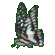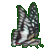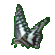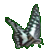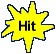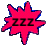Derivative Sum Rule Practice with Powers     Math Help Fun     Game Tips:

- The derivative Sum Rule is used on a sum of terms such as    y = u + v.
In general, the Sum Rule states that the derivative of the sum function    y = u + v  is    y' = u' + v'.
This rule also includes the derivative of the function    y = u - v  , namely    y' = u' - v'.

- For example, the derivative of    y = (x3 + x5)  is    y' = (3x2) + (5x4).

- The derivative of    y = (2x3 + x-5)  is    y' = 6x2 - 5x-6.

- This game also uses the following two rules:

1) The Power Rule for y=xn yields the derivative  dy/dx = nxn-1.
For example, the derivatives of y=x4 and s(t)=t-4 are respectively  dy/dx=4x3 and s'(t)=-4t-5.

2) The derivative rule for a Constant times a Function such as y=cu yields the derivative
dy/dx = c(du/dx).
For example, the derivative of y=8x4 is y'=32x3.

- Note that the three symbols  dy/dx,  y' and  f '(x)  all represent the same derivative of y=f(x).

- Your Game Score is reduced by the number of butterfly hits.

- To slow the game speed repeat tap/click on the word Slider.
- To increase the game speed repeat tap/click on the word Math.
- Speed can also be adjusted with a keyboard's - and + keys.

- Refresh/Reload the web page to restart the game.